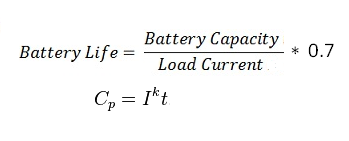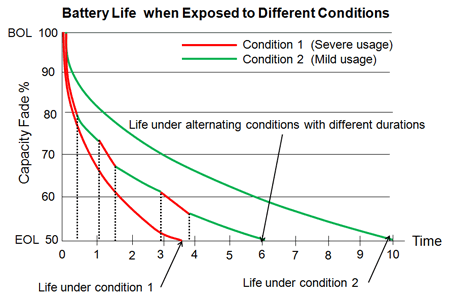# Battery Life Calculator

This Calctown calculator calculates the actual battery life of a lead acid battery.

Peukert's law, presented by the German scientist Wilhelm Peukert in 1897, expresses the capacity of a battery in terms of the rate at which it is discharged. As the rate increases, the battery's available capacity decreases.

If the battery is discharged in a shorter time, with a higher current, the delivered capacity is less. Peukert's law describes a power relationship between the discharge current (normalized to some base rated current) and delivered capacity (normalized to the rated capacity) over some specified range of discharge currents.

#### Result

hrs.
hrs.Click here to view image

Where,

Cp = Capacity of the battery at 1A discharge rate.

I = Actual discharge current (i.e. current drawn from the load).

t = Actual time to discharge the battery (i.e. Battery life)

k = Peukert Constant (= 1.3 for Lead Acid battery).

If the exponent constant k was one, the delivered capacity would be independent of the current.  The Peukert constant varies according to the age of the battery, generally increasing with age. Application at low discharge rates must take into account the battery self-discharge current. At very high currents, practical batteries will give even less capacity than predicted from a fixed exponent. The equation does not account for the effect of temperature on battery capacity.